# Civil Engineering - Applied Mechanics

### Exercise :: Applied Mechanics - Section 5

6.

The reaction at the central support B of the beam ABC hinged at D shown in below figure is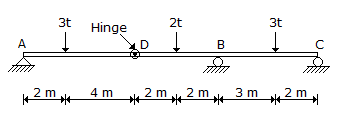A. 2 t B. 5.8 t C. 0.2 t D. 3.5 t.

Explanation:

No answer description available for this question. Let us discuss.

7.

The motion of a bicycle wheel is

 A. translatory B. rotary C. rotary and translatory D. curvilinear

Explanation:

No answer description available for this question. Let us discuss.

8.

The displacement of a particle which moves along a straight line is given by S = 4t3 + 3t2 - 10 where S is in meters and t is in seconds. The time taken by the particle to acquire a velocity of 18 m/sec from rest, is

 A.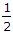sec B. 1 sec C. 1.5 sec D. 1.5 sec.

Explanation:

No answer description available for this question. Let us discuss.

9.

If the resultant of two forces P and Q acting at an angle θ makes an angle α with P, then tan α equals

 A.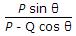B.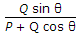C.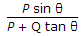D.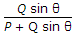Explanation:

No answer description available for this question. Let us discuss.

10.

Pick up the correct statement from the following. The kinetic energy of a body

 A. before impact is equal to that after impact B. before impact is less than that after impact C. before impact is more than that after impact D. remains constant E. none of these.